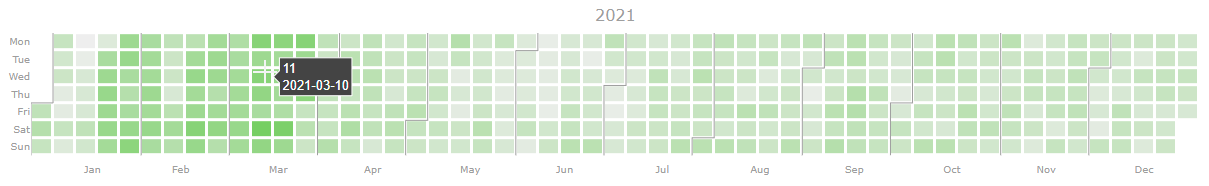{{ message }}

Instantly share code, notes, and snippets.

# bendichter/plotly_activity_chart.py

Last active Apr 27, 2022
This file contains bidirectional Unicode text that may be interpreted or compiled differently than what appears below. To review, open the file in an editor that reveals hidden Unicode characters. Learn more about bidirectional Unicode characters
 # MIT LICENSE import datetime import plotly.graph_objs as go from plotly.subplots import make_subplots import numpy as np import dash_core_components as dcc import dash_html_components as html import dash def display_year( z, year: int = None, month_lines: bool = True, fig=None, row: int = None ): if year is None: year = datetime.datetime.now().year d1 = datetime.date(year, 1, 1) d2 = datetime.date(year, 12, 31) number_of_days = (d2-d1).days + 1 data = np.ones(number_of_days) * np.nan data[:len(z)] = z d1 = datetime.date(year, 1, 1) d2 = datetime.date(year, 12, 31) delta = d2 - d1 month_names = ['Jan', 'Feb', 'Mar', 'Apr', 'May', 'Jun', 'Jul', 'Aug', 'Sep', 'Oct', 'Nov', 'Dec'] month_days = [31, 28, 31, 30, 31, 30, 31, 31, 30, 31, 30, 31] if number_of_days == 366: # leap year month_days = 29 month_positions = (np.cumsum(month_days) - 15)/7 dates_in_year = [d1 + datetime.timedelta(i) for i in range(delta.days+1)] # list with datetimes for each day a year weekdays_in_year = [i.weekday() for i in dates_in_year] # gives [0,1,2,3,4,5,6,0,1,2,3,4,5,6,…] (ticktext in xaxis dict translates this to weekdays weeknumber_of_dates = [] for i in dates_in_year: inferred_week_no = int(i.strftime("%V")) if inferred_week_no >= 52 and i.month == 1: weeknumber_of_dates.append(0) elif inferred_week_no == 1 and i.month == 12: weeknumber_of_dates.append(53) else: weeknumber_of_dates.append(inferred_week_no) text = [str(i) for i in dates_in_year] #gives something like list of strings like ‘2018-01-25’ for each date. Used in data trace to make good hovertext. #4cc417 green #347c17 dark green colorscale=[[False, '#eeeeee'], [True, '#76cf63']] # handle end of year data = [ go.Heatmap( x=weeknumber_of_dates, y=weekdays_in_year, z=data, text=text, hoverinfo='text', xgap=3, # this ygap=3, # and this is used to make the grid-like apperance showscale=False, colorscale=colorscale ) ] if month_lines: kwargs = dict( mode='lines', line=dict( color='#9e9e9e', width=1, ), hoverinfo='skip', ) for date, dow, wkn in zip( dates_in_year, weekdays_in_year, weeknumber_of_dates ): if date.day == 1: data += [ go.Scatter( x=[wkn-.5, wkn-.5], y=[dow-.5, 6.5], **kwargs, ) ] if dow: data += [ go.Scatter( x=[wkn-.5, wkn+.5], y=[dow-.5, dow - .5], **kwargs, ), go.Scatter( x=[wkn+.5, wkn+.5], y=[dow-.5, -.5], **kwargs, ) ] layout = go.Layout( title='activity chart', height=250, yaxis=dict( showline=False, showgrid=False, zeroline=False, tickmode='array', ticktext=['Mon', 'Tue', 'Wed', 'Thu', 'Fri', 'Sat', 'Sun'], tickvals=[0, 1, 2, 3, 4, 5, 6], autorange="reversed", ), xaxis=dict( showline=False, showgrid=False, zeroline=False, tickmode='array', ticktext=month_names, tickvals=month_positions, ), font={'size':10, 'color':'#9e9e9e'}, plot_bgcolor=('#fff'), margin = dict(t=40), showlegend=False, ) if fig is None: fig = go.Figure(data=data, layout=layout) else: fig.add_traces(data, rows=[(row+1)]*len(data), cols=*len(data)) fig.update_layout(layout) fig.update_xaxes(layout['xaxis']) fig.update_yaxes(layout['yaxis']) return fig def display_years(z, years): day_counter = 0 fig = make_subplots(rows=len(years), cols=1, subplot_titles=years) for i, year in enumerate(years): d1 = datetime.date(year, 1, 1) d2 = datetime.date(year, 12, 31) number_of_days = (d2-d1).days + 1 data = z[day_counter : day_counter + number_of_days] display_year(data, year=year, fig=fig, row=i) fig.update_layout(height=250*len(years)) day_counter += number_of_days return fig z = np.random.randint(2, size=(1200,)) display_years(z, (2020, 2021, 2022))

### mihai80 commented Dec 7, 2020

This is great, thank you!

### bayees commented Jan 7, 2022 • edited

I have a correction for your script. The year period should probally go from first date of week 1 until last day of the last week of the year.

`````` d1 = datetime.datetime.strptime(f'{year}-1-1', "%Y-%W-%w").date()
d2 = datetime.datetime.strptime(f'{year}-{datetime.date(year, 12, 28).isocalendar()}-0', "%Y-%W-%w").date()
``````

It depends on how you want to see the offset days, but the current script does not work for 2021 - 2022

### bayees commented Jan 7, 2022 • edited

I made different solution that actually show the whole year now.

``````  weeknumber_of_dates = []
for i in dates_in_year:
inferred_week_no = int(i.strftime("%V"))
if inferred_week_no >= 52 and i.month == 1:
weeknumber_of_dates.append(0)
elif inferred_week_no == 1 and i.month == 12:
weeknumber_of_dates.append(53)
else:
weeknumber_of_dates.append(inferred_week_no)
``````

And change the hardcoded 365 days to a calculate the days of the year since 2020 has 366 days.

``````d1 = datetime.date(year, 1, 1)
d2 = datetime.date(year, 12, 31)

number_of_days = (d2-d1).days + 1
``````

### bendichter commented Jan 10, 2022

thanks @bayees! With your held I improved this gist in the following ways:

• fixed import error
• account for leap years
• fix rendering of last week

### TPhil10 commented Jan 22, 2022

This is great! One question though on line 40, should that be `if number_of_days == 366:` instead of `if number_of_days == 266:`? Leap year would just be added a day to a normal 365 day year, right?

### bendichter commented Jan 24, 2022

@TPhil10 you are absolutely right. Thanks for catching that!

### bweisshaupt commented Mar 17, 2022

Thank you for this great visualization! I like to see the underlying value of a day in the hovertext. Therefore I changed your line 70 to: "hoverinfo='text+z',"### bendichter commented Mar 17, 2022

@bweisshaupt very cool!